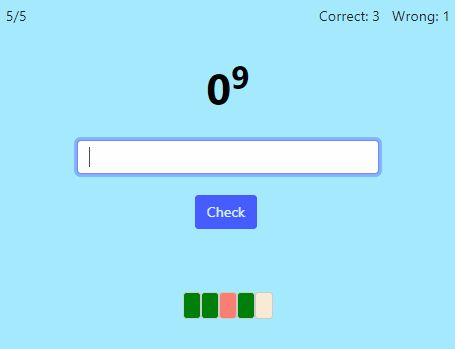# Exponents and Powers of TenOnline practice for grades 5-7

On this page, you can practice with exponents and powers, such as 52 or 106. Students usually learn about exponents in 5th or 6th grade, but 7th grade students can benefit from practicing them too.

An exponent is the little number in this expression: 24. It indicates how many times the base number (in our case 2) is multiplied by itself. So, 24 means 2 × 2 × 2 × 2 = 16.

You can choose to practice either simple expressions with exponents, or expressions where we multiply a power of ten by some factor, or both.

You can choose timed or untimed practice and the number of practice problems.

Screenshot:OR
Practice for a set time: min